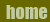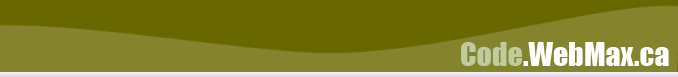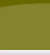This is a simple piece of code to display a bar chart in PHP using GD.

Store the values you wish to chart in an array. The code will size the columns to fit the width and height specified for the graph. The value in \$padding is used to provide a space between each column.

The code in the example returns the graph in PNG format. You can obviously change this to any other format you wish. Just remember that when using JPEG you will experience some loss of sharpness in the image, and it may not be suitable for this type of image application.

```<?php // This array of values is just here for the example.     \$values = array("23","32","35","57","12",                     "3","36","54","32","15",                     "43","24","30"); // Get the total number of columns we are going to plot     \$columns  = count(\$values); // Get the height and width of the final image     \$width = 300;     \$height = 200; // Set the amount of space between each column     \$padding = 5; // Get the width of 1 column     \$column_width = \$width / \$columns ; // Generate the image variables     \$im        = imagecreate(\$width,\$height);     \$gray      = imagecolorallocate (\$im,0xcc,0xcc,0xcc);     \$gray_lite = imagecolorallocate (\$im,0xee,0xee,0xee);     \$gray_dark = imagecolorallocate (\$im,0x7f,0x7f,0x7f);     \$white     = imagecolorallocate (\$im,0xff,0xff,0xff);      // Fill in the background of the image     imagefilledrectangle(\$im,0,0,\$width,\$height,\$white);          \$maxv = 0; // Calculate the maximum value we are going to plot     for(\$i=0;\$i<\$columns;\$i++)\$maxv = max(\$values[\$i],\$maxv); // Now plot each column              for(\$i=0;\$i<\$columns;\$i++)     {         \$column_height = (\$height / 100) * (( \$values[\$i] / \$maxv) *100);         \$x1 = \$i*\$column_width;         \$y1 = \$height-\$column_height;         \$x2 = ((\$i+1)*\$column_width)-\$padding;         \$y2 = \$height;         imagefilledrectangle(\$im,\$x1,\$y1,\$x2,\$y2,\$gray); // This part is just for 3D effect         imageline(\$im,\$x1,\$y1,\$x1,\$y2,\$gray_lite);         imageline(\$im,\$x1,\$y2,\$x2,\$y2,\$gray_lite);         imageline(\$im,\$x2,\$y1,\$x2,\$y2,\$gray_dark);     } // Send the PNG header information. Replace for JPEG or GIF or whatever     header ("Content-type: image/png");     imagepng(\$im); ?>```